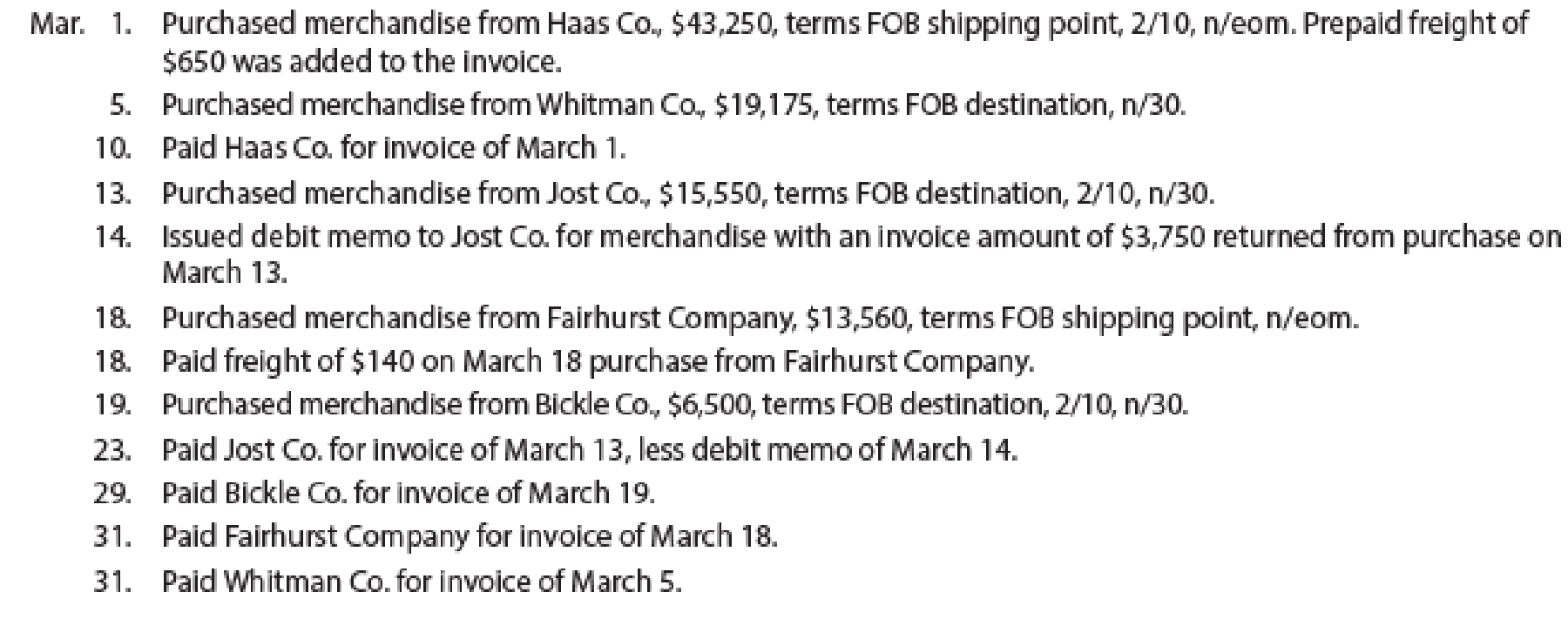Chapter 5, Problem 1PB

Chapter
Section
Textbook Problem

Purchase-related transactions using perpetual inventory systemThe following selected transactions were completed by Niles Co. during March of the current year:InstructionsJournalize the entries to record the transactions of Niles Co. for March.

To determine

Journal entry: Journal is the book of original entry whereby all the financial transactions are recorded in chronological order. Under this method each transaction has two sides, debit side and credit side. Total amount of debit side must be equal to the total amount of credit side. In addition, it is the primary books of accounts for any entity to record the daily transactions and processed further till the presentation of the financial statements.

The following are the rules of debit and credit:

1. 1. Increase in assets and expenses accounts are debited. Decrease in liabilities and stockholders’ equity accounts are debited.
2. 2. Increase in liabilities, revenues, and stockholders’ equity accounts are credited. Decreases in all asset accounts are credited.

Prepare journal entries to record the transactions of Company N during the month of March using perpetual inventory system.

Explanation

Perpetual Inventory System refers to the inventory system that maintains the detailed records of every inventory transactions related to purchases and sales on a continuous basis. It shows the exact on-hand- inventory at any point of time.

Record the journal entry of Company N.

 Date Account Title and Explanation Post Ref. Debit ($) Credit ($) March 1 Inventory 43,035 Accounts payable 43,035 (1) (To record purchase on account)

Table (1)

Working Note:

Calculate the amount of accounts payable.

Purchases = $43,250 Discount percentage = 2% Freight charges =$650

Amount of accounts payable} = [(PurchasesDiscount)+Freight]=[Purchases(Purchases×2%)+Freight][$43,250 – ($43,250×2%)+$650]=$43,250$865+$650=$43,035 (1) • Inventory is an asset and it is increased by$43,035. Therefore, debit Inventory account with $43,035. • Accounts payable is a liability and it is increased by$43,035. Therefore, credit accounts payable account with $43,035. Record the journal entry of Company N.  Date Account Title and Explanation Post Ref. Debit ($) Credit ($) March 5 Inventory 19,175 Accounts payable 19,175 (To record purchase on account) Table (2) • Inventory is an asset and it is increased by$19,175. Therefore, debit Inventory account with $19,175. • Accounts payable is a liability and it is increased by$19,175. Therefore, credit accounts payable account with $19,175. Record the journal entry of Company N.  Date Account Title and Explanation Post Ref. Debit ($) Credit ($) March 10 Accounts payable 43,035 Cash 43,035 (To record the payment against accounts payable) Table (3) • Accounts payable is a liability and it is decreased by$43,035. Therefore, debit accounts payable account with $43,035. • Cash is an asset and it is decreased by$43,035. Therefore, credit cash account with $43,035. Record the journal entry of Company N.  Date Account Title and Explanation Post Ref. Debit ($) Credit ($) March 13 Inventory 15,239 Accounts payable 15,239 (2) (To record purchase on account) Table (4) Working Note: Calculate the amount of accounts payable. Purchases =$15,550

Discount percentage = 2%

Amount of accounts payable} = (PurchasesDiscount)=Purchases(Purchases×2%)= $15,550 – ($15,550×2%)= $15,550$311=$15,239 (2) • Inventory is an asset and it is increased by$15,239. Therefore, debit Inventory account with $15,239. • Accounts payable is a liability and it is increased by$15,239. Therefore, credit accounts payable account with $15,239. Record the journal entry of Company N.  Date Account Title and Explanation Post Ref. Debit ($) Credit ($) March 14 Accounts payable 3,675 (3) Inventory 3,675 (To record purchase return) Table (5) Working Note: Calculate the amount of accounts payable. Purchases return =$3,750

Discount percentage = 2%

Amount of accounts payable} = (Purchases returnDiscount)=Purchases return(Purchases return×2%)= $3,750 – ($3,750×2%)= $3,750$75=$3,675 (3) • Accounts payable is a liability and it is decreased by$3,675

Still sussing out bartleby?

Check out a sample textbook solution.

See a sample solution

The Solution to Your Study Problems

Bartleby provides explanations to thousands of textbook problems written by our experts, many with advanced degrees!

Get Started

Find more solutions based on key concepts